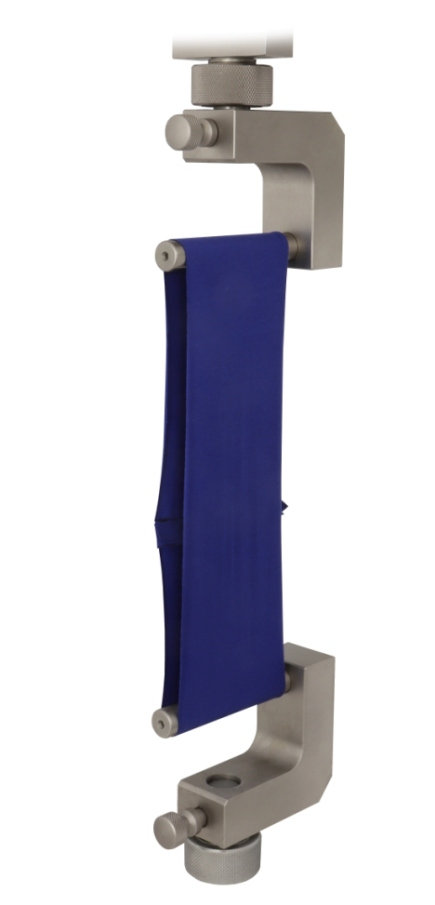## Standard Testing of Tension and Elongation of Elastic Fabrics (Elastomers)###### ​

ASTM D4964 involves comprehension of the equation L = (E X C) / 200

Where:

E is % elongation

C is loop circumference

L = extension;

( or Length, or Mathematical Difference from origin.)

This equation is derived and therefore predicated upon the mutal understandings

a. loop tension is defined as the total tension at any specified extension that is exerted on a specimen in a loop formation,

and

b. elongation is understood as the ratio of the extension of a material to the length of the material prior to stretching.

###### Geometry:

To test the equation appropriately, one may rely on universal grip co band clamp attached to universal testing machine with appropriate executable software pre-installed:

Recommended is

constant rate of extension tensile test machnie.

ie. band clamps, and elastomer speciemen

For "Thin" test subjects it is recommended to cut 5 plus specimens of the intended elastomer example.

###### Solution:

Simple solution finding here, as we follow equation L = (E X C) / 200 and plot on a pre-programmed CRE-type tensile testing machine (a UTM.)

l = (e x c) / 200

remember elongation as L, distance from 0. and t time in seconds to develop a graph like

We test elongation at desired specifications 30%, 50%, and 70%. Generally we test each spec. 3 times.

Analysis:

###### Similar Specifications:

"SimSpecs" may involve a global study of elastomers, aforementioned Youn'gs Modulous from physics and Also definitly read the following ASTM  on wet paper shearing.

https://www.universalgripco.com/astm-d378

In Stock Item!